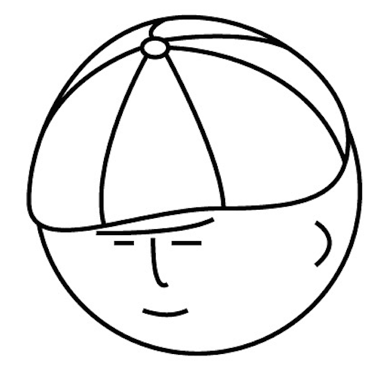# マツシタのお勉強メモ

#### マツシタのお勉強

##### welcome to my engineering blog
###### Hacker Rank# Problem

Implement a function to check if a binary tree is a binary search tree

# Solution

There are some solutions to solve this problem. I’ll introduce the all solution which includes I came up with. First Solution is using a range (min, max)

#### The Min / Max Solution

This solution is that we keep a range at each node. As traversing the tree, we update the range that the value of node can be possible. In first node (root), we set the range as (min = Int_Min, max = Int_max). And if we traverse to left, we have to change the max to the value of the current node. And if we traverse to right, we have to change the min to the value of the current node.

This solution takes O(N) times and space complexity is O(log N) (log N is the depth of the tree). This is best solution.

#### In-Oder Traversal Solution

A binary search tree can be a sorted array by in-oder traversal. So we make the array and the check if the array is sorted.

But this solution can not handle duplicate values int the tree values. In binary search tree, if left.value <= current.value, it is valid. But if current.value <= right.value, it is invalid. This solution can not distinguish the difference.